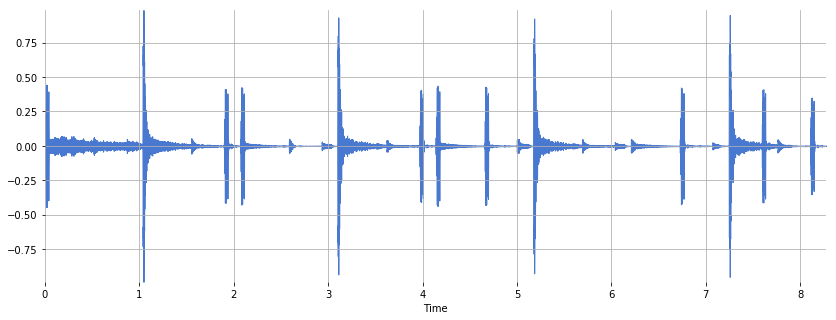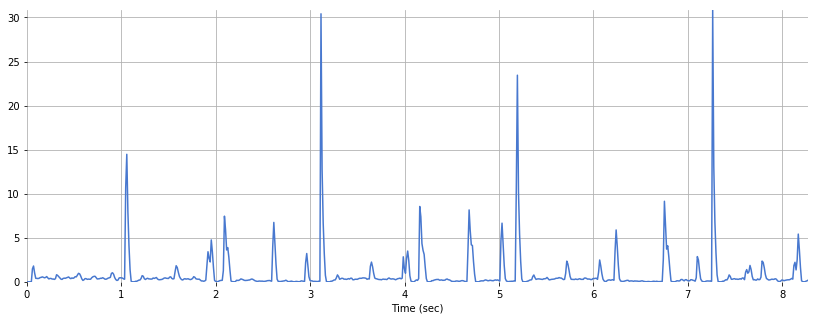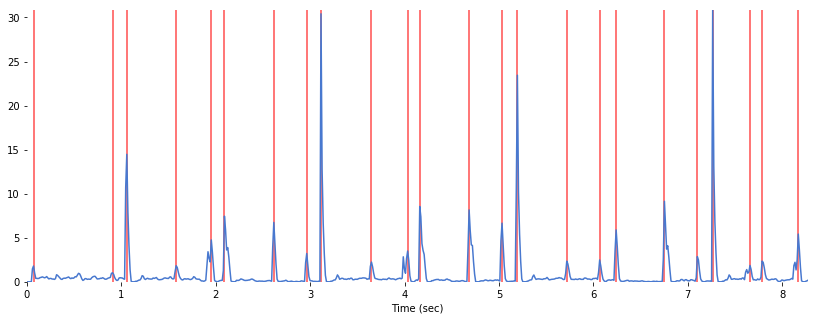In :
%matplotlib inline
import matplotlib.pyplot as plt, IPython.display as ipd, numpy
import librosa, librosa.display
import stanford_mir; stanford_mir.init()


# Peak Picking¶

Peak picking is the act of locating peaks in a signal. For example, in onset detection, we may want to find peaks in a novelty function. These peaks would correspond to the musical onsets.

Let's load an example audio file.

In :
x, sr = librosa.load('audio/58bpm.wav')

In :
print(x.shape, sr)

(182464,) 22050


Listen to the audio file:

In :
ipd.Audio(x, rate=sr)

Out:

Plot the signal:

In :
plt.figure(figsize=(14, 5))
librosa.display.waveplot(x, sr=sr)

Out:
<matplotlib.collections.PolyCollection at 0x119ac5ba8>Compute an onset envelope:

In :
hop_length = 256
onset_envelope = librosa.onset.onset_strength(x, sr=sr, hop_length=hop_length)

In :
onset_envelope.shape

Out:
(713,)

Generate a time variable:

In :
N = len(x)
T = N/float(sr)
t = numpy.linspace(0, T, len(onset_envelope))


Plot the onset envelope:

In :
plt.figure(figsize=(14, 5))
plt.plot(t, onset_envelope)
plt.xlabel('Time (sec)')
plt.xlim(xmin=0)
plt.ylim(0)

Out:
(0, 30.846780503223634)In this onset strength envelope, we clearly see many peaks. Some correspond to onsets, and others don't. How do we create peak picker that will detect true peaks while avoiding unwanted spurious peaks?

librosa.util has a peak_pick method. We can control the parameters based upon our signal. Let's see how it works:

def peak_pick(x, pre_max, post_max, pre_avg, post_avg, delta, wait):
'''Uses a flexible heuristic to pick peaks in a signal.

A sample n is selected as a peak if the corresponding x[n]
fulfills the following three conditions:

1. x[n] == max(x[n - pre_max:n + post_max])
2. x[n] >= mean(x[n - pre_avg:n + post_avg]) + delta
3. n - previous_n > wait

where previous_n is the last sample picked as a peak (greedily).

Get the frame indices of the peaks:

In :
onset_frames = librosa.util.peak_pick(onset_envelope, 7, 7, 7, 7, 0.5, 5)

In :
onset_frames

Out:
array([  6,  78,  91, 136, 168, 180, 225, 255, 268, 314, 347, 358, 403,
433, 447, 492, 522, 537, 581, 611, 625, 659, 670, 703])

Plot the onset envelope along with the detected peaks:

In :
plt.figure(figsize=(14, 5))
plt.plot(t, onset_envelope)
plt.grid(False)
plt.vlines(t[onset_frames], 0, onset_envelope.max(), color='r', alpha=0.7)
plt.xlabel('Time (sec)')
plt.xlim(0, T)
plt.ylim(0)

Out:
(0, 30.846780503223634)Superimpose a click track upon the original:

In :
clicks = librosa.clicks(frames=onset_frames, sr=22050, hop_length=hop_length, length=N)

In :
ipd.Audio(x+clicks, rate=sr)

Out: